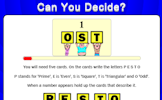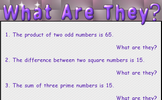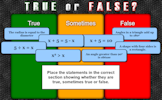# Prime numbers and proof

### Term 3 starting in week 11 :: Estimated time: 2 weeks

• Find and use multiples
• Identify factors of numbers and expressions
• Recognise and identify prime numbers
• Recognise square and triangular numbers
• Find common factors of a set of numbers including the HCF
• Find common multiples of a set of numbers including the LCM
• Write a number as a product of its prime factors
• Make and test conjectures
• Use counterexamples to disprove a conjecture

For higher-attaining pupils:

• Use a Venn diagram to calculate the HCF and LCM

This page should remember your ticks from one visit to the next for a period of time. It does this by using Local Storage so the information is saved only on the computer you are working on right now.

## Lesson Starters

Here are some suggestions for whole-class, projectable resources which can be used at the beginnings of each lesson in this block.

### 1st Lesson#### Can You Decide?

Recognise odd, even, square, prime and triangular numbers.

### 2nd Lesson#### What are they?

A starter about sums, products, differences, ratios, square and prime numbers.

### 3rd Lesson#### Abundant Buses

A game based around the concept of factors and abundant numbers.

### 4th Lesson#### True or False?

An activity designed to address common misconceptions.

### 5th Lesson#### Hotel Digital

A puzzle about the lifts in a hotel which serve floors based on the day of the week.

### 6th Lesson#### Flabbergasted

If each number in a sequence must be a factor or multiple of the previous number what is the longest sequence that can be made from the given numbers?

Some of the Starters above are to reinforce concepts learnt, others are to introduce new ideas while others are on unrelated topics designed for retrieval practice or and opportunity to develop problem-solving skills.

White Rose ResourcesEnd of block assessments provide a quick progress check at the end of each block of learning to make sure students have understood the content covered. This Scheme of Learning was produced by White Rose Maths and is used here with permission granted on 30th June 2021.For All: